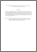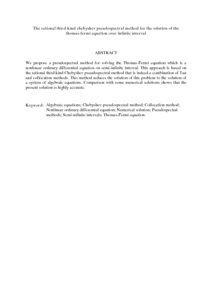# The rational third-kind chebyshev pseudospectral method for the solution of the thomas-fermi equation over infinite interval

## Citation

Kajani, Majid Tavassoli and Kilicman, Adem and Maleki, Mohammad (2013) The rational third-kind chebyshev pseudospectral method for the solution of the thomas-fermi equation over infinite interval. Mathematical Problems in Engineering, 2013. art. no. 537810. pp. 1-6. ISSN 1024-123X

## Abstract

We propose a pseudospectral method for solving the Thomas-Fermi equation which is a nonlinear ordinary differential equation on semi-infinite interval. This approach is based on the rational third-kind Chebyshev pseudospectral method that is indeed a combination of Tau and collocation methods. This method reduces the solution of this problem to the solution of a system of algebraic equations. Comparison with some numerical solutions shows that the present solution is highly accurate.Preview
PDF (Abstract)
The rational third.pdfView Item